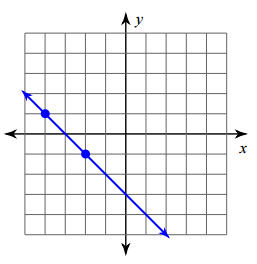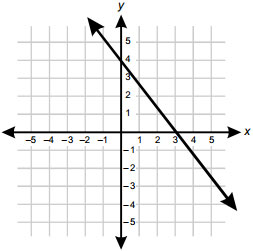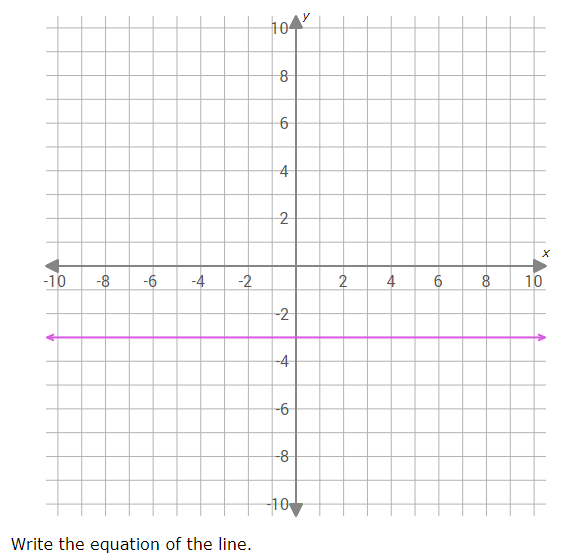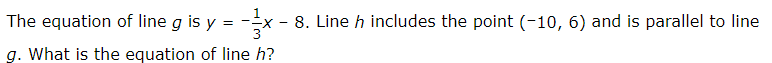# Functions and Linear Equations

This set of MCQs helps you brush up on important math topics and prepare you to dive into skill practice.

Start Quiz

Find the slope of the line that passes through the points (2, 4) and (6, 12).

-1/2

2

-2

1/2

Find the slope of the line.2

-1

1

-2

Bob has \$150 in his savings account and saves \$40 per month. Write an equation to model this scenario.

y = 40x + 150

y = 150x + 150

y = 150x + 40

y = 40x + 15

What is the y-intercept of the following graph?(-4,4)

(0,3)

(0,4)

(0,2)

A man is climbing down from 100 feet high descending 30 feet per minute. Write an equation to model this scenario.

30-100x

100-3x

100+30x

100-30x

Is the graph of this equation a horizontal or vertical line? y=–9

Vertical

Horizontaly=–3

x=–3

y=3

–3y = 1/3x + 8/3

y = –1/3x - 8/3

y = –1/3x + 8/3

y = –1/3x - 4/3

Quiz/Test Summary
Title: Functions and Linear Equations
Questions: 8
Contributed by: